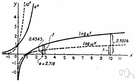# exponential function

(redirected from Exponential functions)
Also found in: Thesaurus, Encyclopedia.
ThesaurusAntonymsRelated WordsSynonymsLegend:
 Noun 1exponential function - a function in which an independent variable appears as an exponentexponentialfunction, mapping, mathematical function, single-valued function, map - (mathematics) a mathematical relation such that each element of a given set (the domain of the function) is associated with an element of another set (the range of the function)
Based on WordNet 3.0, Farlex clipart collection. © 2003-2012 Princeton University, Farlex Inc.
References in periodicals archive ?
In short, powerful exponential functions that underpin the speedy expansion of the universe also explain the effectiveness of the genetic algorithm and evolution.
Since using linear functions did not yield the expected results when it came to HDR compression, the focus shifted towards logarithmic and exponential functions.
Thus, the Mittag-Leffler functions can be considered as the analogy of exponential functions for fractional differential operators, since they are the sum of all basis elements.
If the inner solution is an exponential function, then the proposed schemes give the exact solution since the formulae used for the first and second derivative are exact for exponential functions.
Akter and Akbar  utilized the modified simple equation method to obtain exact solutions of (11) which were written as fractions of exponential functions. They can be transformed into the tanh and coth functions for which the arbitrary constants are selected appropriately as shown in their paper.
Hyperbolic, logarithmic, power, and exponential functions were used as the prediction models for the variation of settlement with time for both road shoulder and driveway, as shown in Figures 7 and 8.
Narala and Reddy (2012) focused on growth and instability in cultivated area, production and productivity of cotton for the period 1951 - 2011 in India through exponential functions. Reddy et al.
4: Fitting of the semi-major axis values with two different types of exponential functions, i.e., (a) f (n) = [alpha] + [beta][2.sup.n] and (b) f (n) = [alpha] [exp.sup.[beta]n].
There are studies where exponential functions were fitted to the experimental responses [7, 21] and others where a sigmoid function was preferred over the exponential [22, 23], and in some studies the response was directly analyzed or was fitted to other functions [9, 16].
(2) The exponential functions for fresh concrete ([f.sub.ts] = 0.19 [f.sub.cu.sup.3/4], [f.sub.ts] = 0.439 [f.sub.cu.sup.0.55], [f.sub.ts] = 1.34[([f.sub.cu]/10).sup.2/3], [f.sub.ts] = 0.28[f.sub.cu.sup.2/3], and [f.sub.ts] = 0.49[f.sub.cu.sup.0.5]) proposed by building guidelines or literatures only roughly describe the overall tendency of compressive-tensile strength relationship for deteriorated concrete.
Specifically, the sinusoidal, linear, and the exponential functions are used to simulate echo intensity, and then the accumulative rainfalls for both stratiform and convective precipitation are calculated with these two methods
Moreover, the obtained solution can be extended for a wider class of periodic parametric functions including all the functions which can be expressed by the algebraic sum of exponential functions or can be approximated by such functions with sufficiently small error.

Site: Follow: Share:
Open / Close